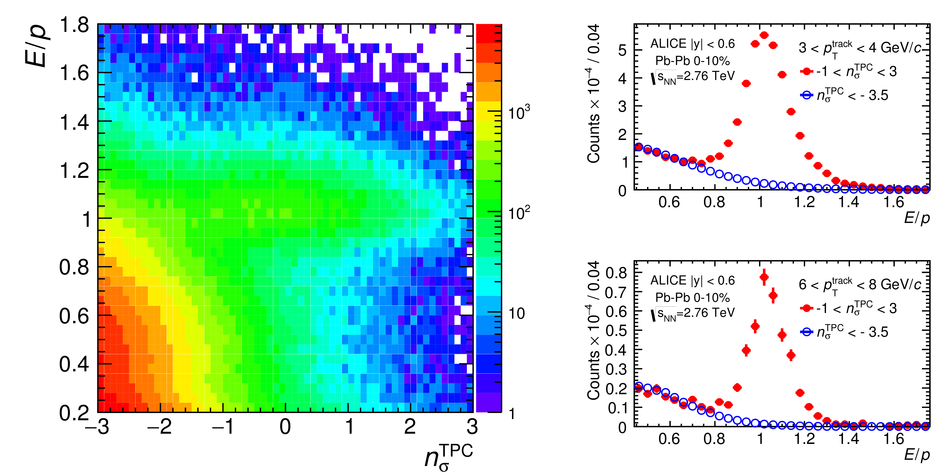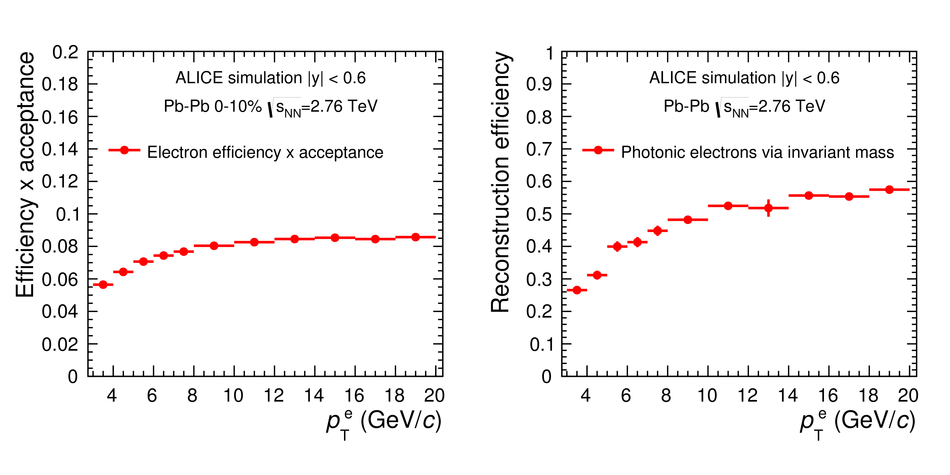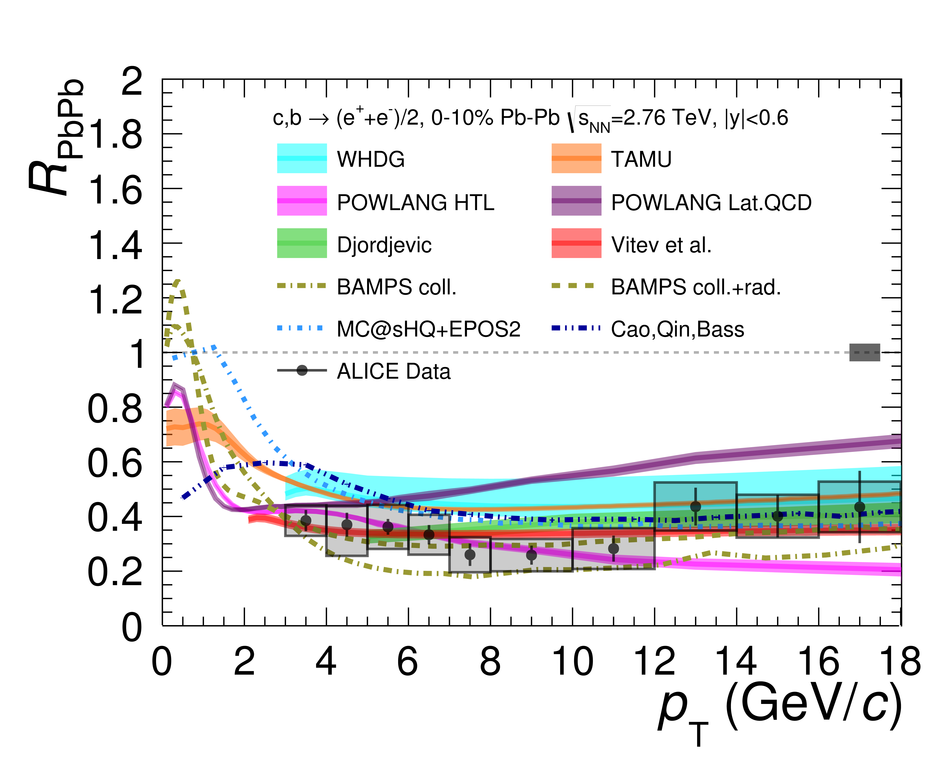Measurement of the production of high-$p_{\rm T}$ electrons from heavy-flavour hadron decays in Pb-Pb collisions at $\sqrt{s_{\rm NN}}$ = 2.76 TeV

Electrons from heavy-flavour hadron decays (charm and beauty) were measured with the ALICE detector in Pb-Pb collisions at a centre-of-mass of energy $\sqrt{s_{\rm NN}}=2.76$ TeV. The transverse momentum ($p_{\rm T}$) differential production yields at mid-rapidity were used to calculate the nuclear modification factor $R_{\rm AA}$ in the interval $3<~p_{\rm T}<~ 18$ GeV/$c$. The $R_{\rm AA}$ shows a strong suppression compared to binary scaling of pp collisions at the same energy (up to a factor of 4) in the 10% most central Pb-Pb collisions. There is a centrality trend of suppression, and a weaker suppression (down to a factor of 2) in semi-peripheral (50-80%) collisions is observed. The suppression of electrons in this broad $p_{\rm T}$ interval indicates that both charm and beauty quarks lose energy when they traverse the hot medium formed in Pb-Pb collisions at LHC.

Figures

Figure 1

 Trigger turn-on curves: the ratio of inclusive electrons in EMCal triggered events to minimum-bias events as a function of associated track $\pt$ in centrality bins between 0% and 50%. The lower right panel shows a similar ratio obtained with EMCal clusters for centrality 50-80%. The $\pt$ from which the spectra from the minimum-bias trigger to the EMCal trigger are used are indicated with black dashed lines. The scaling factors which were obtained by fits (red lines) are summarized in Table 1.Figure 2

 $\bf{Left:}$ The ratio of $E/p$ as a function of $n_{\sigma}^{\rm{TPC}}$ in 10% most central Pb-Pb events ($p_{\rm{T}}>$ 3 GeV/$c$), where $p$ is the charged particle momentum, $E$ is the matched EMCal cluster energy, and $\sigma^{\mathrm{TPC}}$ is the resolution on the energy loss in the TPC gas expected for electrons. $\bf{Right:}$ $E/p$ for electrons in two transverse momentum ranges. The blue open symbols shows the hadron contamination $--$ an $E/p$ distribution for particles 3.5 $\sigma$ away from the mean of the true electron ${\rm TPC}$-d$E$/d$x$ distribution normalized to the electron $E/p$ at small values of the ratio (away from the electron signal).Figure 3

 $\bf{Left:}$ Product of detector acceptance and reconstruction efficiency for inclusive electrons as a function of the electron $\pt$. The statistical uncertainty is smaller than the size of the points. $\bf{Right:}$ Photonic electron reconstruction efficiency via invariant mass ($\varepsilon_{e^{\gamma}}$) as a function of $\pt$ of the electron.Figure 4

 Differential yields of electrons from semi-leptonic decays of heavy-flavour hadrons in classes of centrality of Pb-Pb collisions at $\sqrt{s_{\rm NN}}$ = 2.76 TeV.Figure 5

 $R_{\rm {PbPb}}$ of electrons from heavy-flavour hadron decays in centrality bins of Pb-Pb collisions at $\sqrt{s_{\rm NN}}$ = 2.76 TeV. The solid band at $R_{\rm {PbPb}}=1$ brackets the uncertainty on the average nuclear overlap function ($\langle T_{\rm{AA}} \rangle$)Figure 6

 $\bf{Left:}$ $R_{\rm {PbPb}}$ of electrons and muons  from heavy-flavour hadron decays in 10% most central Pb-Pb collisions shown together with $R_{\rm {pPb}}$ of electrons from minimum bias proton-lead collisions at $\sqrt{s_{\rm NN}}$ = 5.2 TeV . $\bf{Right:}$ $R_{\rm {PbPb}}$ of electrons in semi-peripheral Pb-Pb collisions (50-80% selection for electrons and 40-80% for muons.Figure 7

 $R_{\rm {PbPb}}$ of electrons from heavy-flavour hadron decays measured in 10% most central Pb-Pb collisions at $\sqrt{s_{\rm NN}}$ = 2.76 TeV compared to various theoretical calculations [86-98].